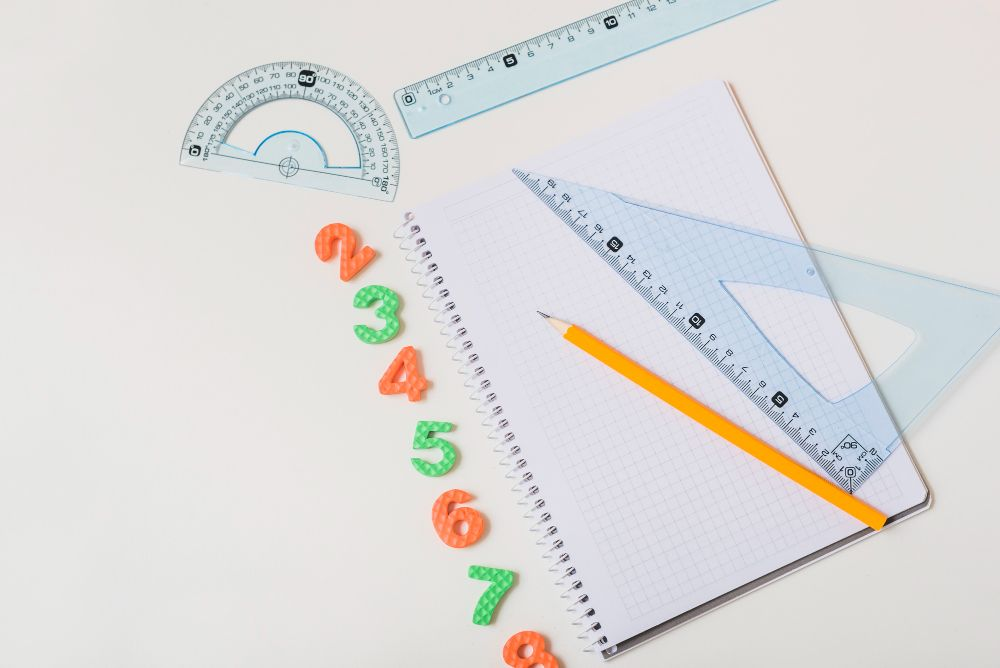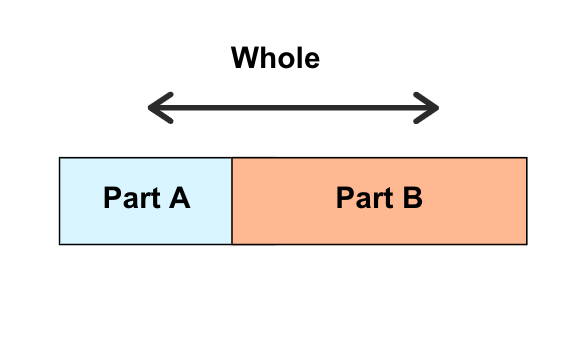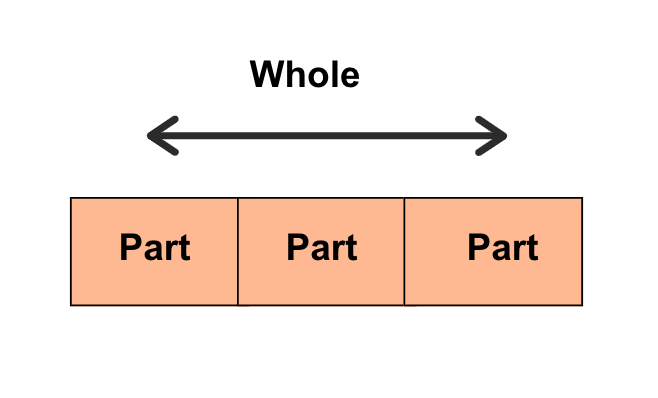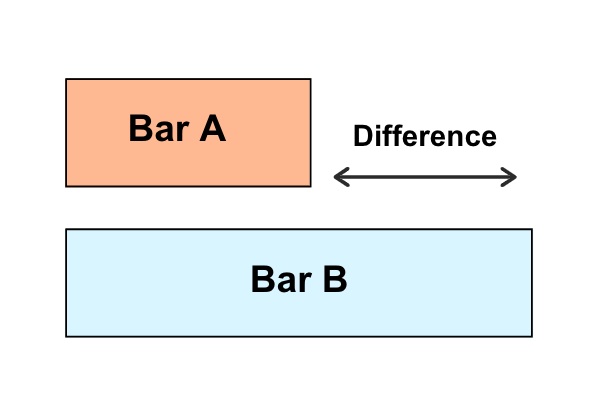If your child is in primary school, then they should be aware of these bar model examples!

Bar models are a common method that students use to visualize and solve math problems. The Singapore bar model method is probably something you’ve heard about. They essentially represent the same meaning!

These models are typically rectangular shaped, much like a bar, with lines separating its interior. The bar represents a total value whereas the divided shapes inside the bar represent parts that make up the total.

Bar models can also represent multiple math concepts, from addition and subtraction to multiplication and division!

Whenever students are confused over how to solve a math problem, they can draw a bar model that represents the problem. From simple math operations to complex worded problems, students can use the bar model problem solving route to find its solutions!

In this article, we’ll go through 3 types of bar model examples that are commonly used in Singapore mathBy being aware of these examples, students can recognize which bar model to use to solve certain math problems!### Part-Whole Model

This model is where parts within the bar represent a total value or a whole.Based on the illustration, we can deduce the part-whole model has two main math operations:

• Part A = Whole – Part B
• Whole = Part A + Part B

Therefore, students can use this bar model to solve math problems involving addition and subtraction.

You can also use this model to solve word problems involving fractions and percentages!

### Equal Parts Model

The equal parts model consists of multiple equal parts that together form a whole.

This model is similar to the part-whole model, except that it can only be used when there are equal parts.Based on the illustration, we can deduce the part-whole model has three main math operations:

• Number of Parts = Whole / Part
• Part = Whole / Number of Parts
• Whole = Number of Parts x Parts

Since the equal parts model doesn’t allow for uneven parts, students can use this model to solve problems involving multiplication and division.

### Comparison Model

Finally, the comparison model involves two bar models in math, emphasizing the difference between them.Unlike the previous bar models, the comparison bar model doesn’t involve any parts inside the bar. Instead, each bar represents a single value.

There are three main operations involved in this bar model:

• Bar A = Bar B – Difference
• Bar B = Bar A – Difference
• Difference = Bar A – Bar B

Much like the part-whole model, the comparison model also involves addition and subtraction.

Students can use this bar model example when they encounter ratio and proportion math problems!

### Practice math using bar models with Practicle!

Bar models can be incredibly useful in visualizing math problems, and it makes children do their problem solving much easier!

If your child needs more practice with them, you can consider utilizing math worksheets on bar models! Students typically receive these bar model worksheets from 3rd grade or Primary 3.

You can also use Practicle’s math learning game to become more familiar with these models!

Practicle provides a gamified math learning experience for primary school students. We use AI math tutors and exciting gaming features to make learning math much more fun for children!

You can try the experience yourself using our 7-day free trial today!